# How To Graph A Function & Its Inverse (4 Key Steps)

Graphing functions comes up all the time in algebra, pre-calculus, calculus, and other subjects.  However, it also helps to know how to graph the inverse of a function.

So, how do you graph a function and its inverse?  First, identify the type of function that f(x) represents (for example, linear). Next, make a table for f(x) with two columns: x & y values. Then, graph f(x) by plotting points and using the shape of the function. Finally, graph the inverse f-1(x) by switching x & y values from the graph of f(x).

Of course, some functions do not have inverses (a function must be one=to-one, passing the horizontal line test, to have an inverse).  In those cases, we won’t be able to graph an inverse function.

In this article, we’ll talk about the 4 key steps to graph a function and its inverse.  We’ll also look at some examples for common functions to make the process clear.

Let’s get started.

## How To Graph A Function & Its Inverse

There are 4 key steps if you want to graph a function and its inverse:

• 1. Identify the type of function – a function f(x) could be linear, exponential, a trig function, or something else.  This step will help you to draw the function later.  When you have an idea of what the function looks like, you will know if you made a mistake when graphing or in your table of values in the next step.
• 2. Create a table of values – a table of values should have two columns: one for x values (inputs) and one for y values (outputs).  Choose a good sample of x values and plug them into f(x) to get y values.  This table will help you to sketch the function after you have a few points.
• 3. Graph the function – to graph f(x), we start by plotting the points from our table in step 2. After this is done, we can sketch the general shape of the graph to connect the points, based on the function type we identified in step 1.
• 4. Graph the inverse – to graph f-1(x), we start by switching x and y values for the points from our table in step 2.  So, the point (2, 5) on the graph of f(x) would give us the point (5, 2) on the graph of f-1(x).

Remember that we can also find the equation for the inverse function by taking the equation y = f(x), switching x and y, then solving for y.  We can then just graph f-1(x) from this equation.

When we graph a function f(x) and its inverse, they will look like mirror images across the line y = x. This makes sense, since the definition of an inverse tells us that f(f-1(x)) = f-1(f(x)) = x.

Also, keep in mind that the domain of the inverse f-1(x) is the range of the function f(x).  Likewise, the range of the inverse f-1(x) is the domain of the function f(x).

Let’s take a look at some examples to show how this process works.  We’ll start with a simple function: a line.

### Example 1: Linear Function Graph & Inverse Graph

Let’s say we have the linear function f(x) = 2x + 6.

For our first step, we identify this as a linear function (it has the form y = mx + b).

For our second step, we create a table of values for this function (shown below).

For our third step, we graph the function by using the points in our table and the general shape of the graph (pictured below).

For our fourth step, we graph the inverse by switching the x and y coordinates for each point in our table (pictured below).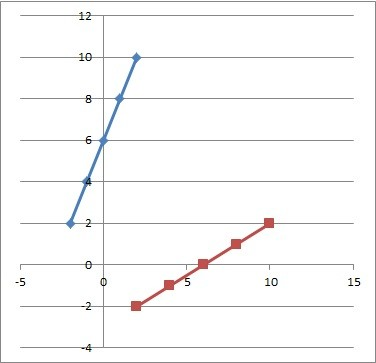The graph of the linear function f(x) = 2x + 6 (blue) and its inverse, f-1(x) = (x – 6) / 2 (red).

To find the equation for the inverse function, we switch x and y, then solve for y:

• f(x) = 2x + 6  [original function]
• y = 2x + 6  [use y instead of f(x)]
• x = 2y + 6  [switch x and y]
• x – 6 = 2y
• (x – 6) / 2 = y

So, we get f-1(x) = (x – 6) / 2, or f-1(x) = (x / 2) – 3.

We can check our work by composing f(x) and f-1(x) to show that f(f-1(x)) = x and f-1(f(x)) = x.

### Example 2: Absolute Value Function Graph & Inverse Graph

Let’s say we have the absolute function f(x) = |x|.

For our first step, we identify this as a piecewise function with two parts: f(x) = x for x >= 0, and f(x) = -x for x < 0 (both parts are linear).

For our second step, we create a table of values for this function (shown below).

For our third step, we graph the function by using the points in our table and the general shape of the graph (pictured below).

Looking at this graph, we can see that it fails the horizontal line test.  For example, we can draw a line y = 2 that intersects the graph at x = -2 and x = 2.

This means that the graph is not one-to-one, and so it does not have an inverse.

### Example 3: Cubic Function Graph & Inverse Graph

Let’s say we have the cubic function f(x) = x3 – 8.

For our first step, we identify this as a cubic function (it has the form y = ax3 + bx2 + cx + d, with b and c zero).

For our second step, we create a table of values for this function (shown below).

For our third step, we graph the function by using the points in our table and the general shape of the graph (pictured below).

For our fourth step, we graph the inverse by switching the x and y coordinates for each point in our table (pictured below).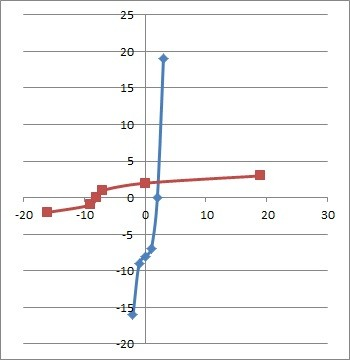The graph of the cubic function f(x) = f(x) = x3 – 8 (blue) and its inverse, f-1(x) = (x + 8)1/3 (red).

To find the equation for the inverse function, we switch x and y, then solve for y:

• f(x) = x3 – 8  [original function]
• y = x3 – 8  [use y instead of f(x)]
• x = y3 – 8  [switch x and y]
• x + 8 = y3
• (x + 8)1/3 = y

So, we get f-1(x) = (x + 8)1/3 or f-1(x) = 3√(x + 8).

We can check our work by composing f(x) and f-1(x) to show that f((f-1(x)) = x and f-1(f(x)) = x.

### Example 4: Exponential Function Graph & Inverse Graph

Let’s say we have the exponential function f(x) = 2x.

For our first step, we identify this as an exponential function (it has the form y = bx, where b is a positive constant).

For our second step, we create a table of values for this function (shown below).

For our third step, we graph the function by using the points in our table and the general shape of the graph (pictured below).

For our fourth step, we graph the inverse by switching the x and y coordinates for each point in our table (pictured below).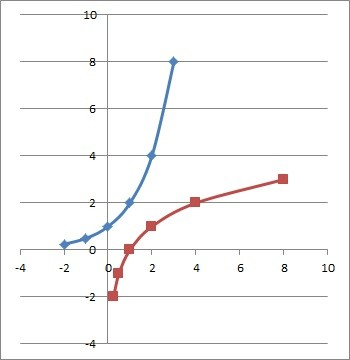The graph of the exponential function f(x) = f(x) = 2x (blue) and its inverse, f-1(x) = log2(x) (red).

To find the equation for the inverse function, we switch x and y, then solve for y:

• f(x) = 2x  [original function]
• y = 2x  [use y instead of f(x)]
• x = 2y  [switch x and y]
• log2(x) = y

So, we get f-1(x) = log2(x).

We can check our work by composing f(x) and f-1(x) to show that f((f-1(x)) = x and f-1(f(x)) = x.

Note: an exponential function ax has an inverse of loga(x), and vice versa.

### Example 5: Trig Function Graph & Inverse Graph (Sine)

Let’s say we have the trigonometric function f(x) = sin(x).

For our first step, we identify this as a trigonometric function (this means that it is repeating).

For our second step, we create a table of values for this function (shown below).

For our third step, we graph the function by using the points in our table and the general shape of the graph (pictured below).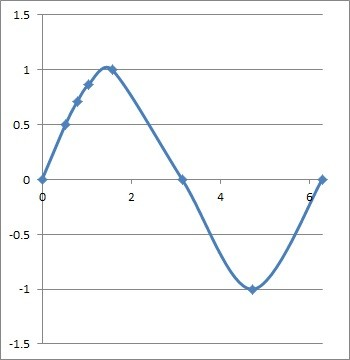The graph of the trigonometric function f(x) = sin(x), showing only one period from x = 0 to x = 2π (360 degrees).

Note that this function is not one-to-one on the domain of real numbers.  If we restrict the domain to one period of the function (0 <= x <= 2π), then we can find the inverse.

For our fourth step, we graph the inverse by switching the x and y coordinates for each point in our table (pictured below).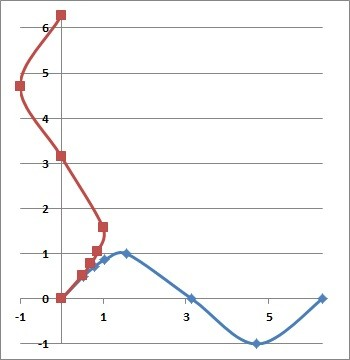The graph of the trigonometric function f(x) = sin(x) (blue) and its inverse, f-1(x) = arcsin(x) (red).

The inverse function is written as f-1(x) = sin-1(x) or f-1(x) = arcsin(x).

Note: the graph for cosine would look similar, with a shift 90 degrees (π/2 radians) to the left.  The inverse of cosine would look similar to the inverse of sine, with a shift of 90 degrees (π/2 radians) down.

## Conclusion

Now you know how to graph a function and its inverse with a simple two-column table.  You also know what the inverses of some common functions look like when graphed.